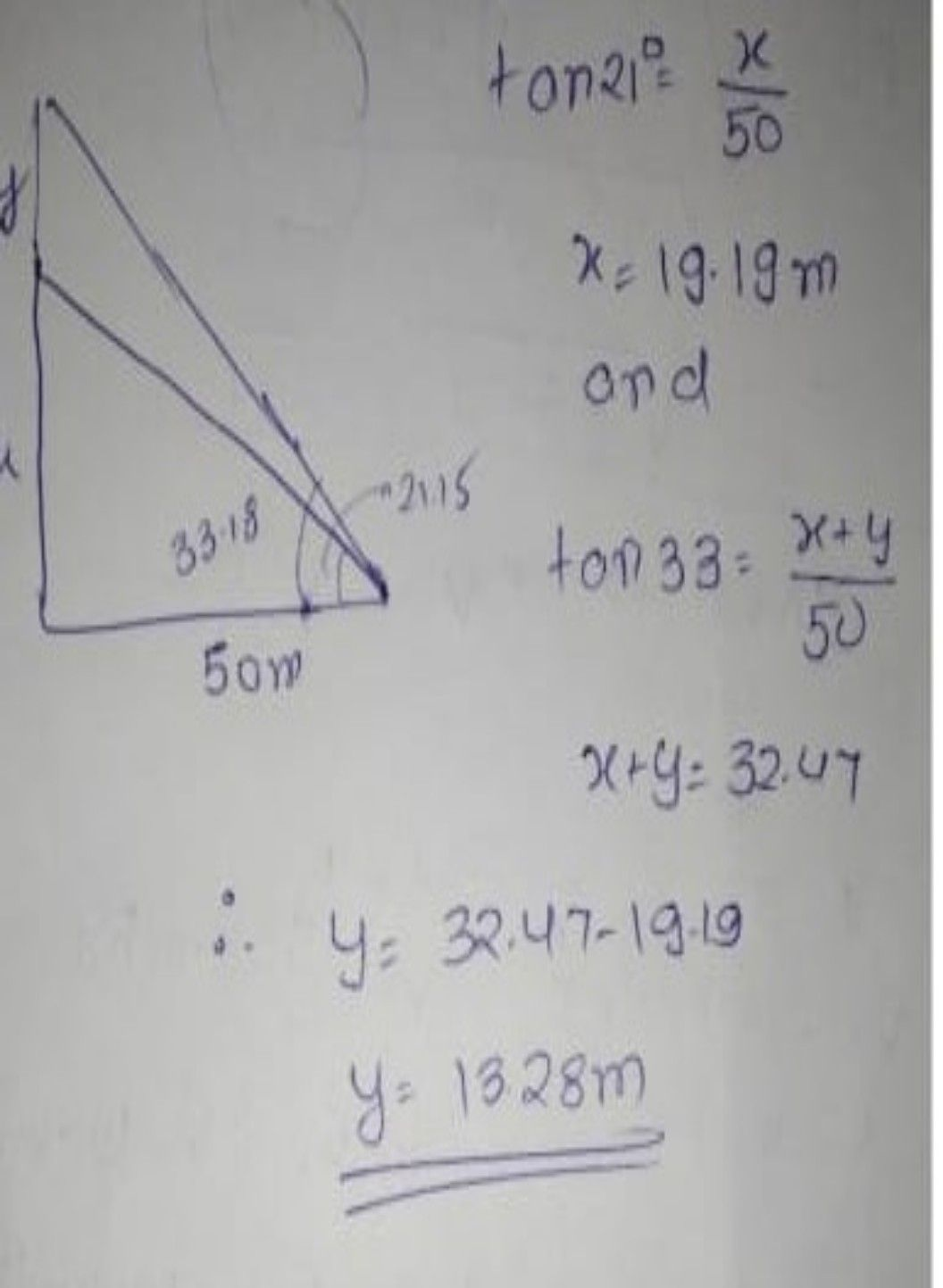Symbol
Problem$11At0$ $10$ flag is fixed vertically at the top of $a$ building, from a point located $60$ $m$ From the base of the building, the elevation angles to the foot $\times 010to$ the top of the to the flag are $21A$ $\begin{cases} ° \\ 50\right)y35<3\right) \end{cases}$ $10A$ $\left(2\right)$ Find the measure of the to Measure of the flagpole is $128115m2Da21250\pi$